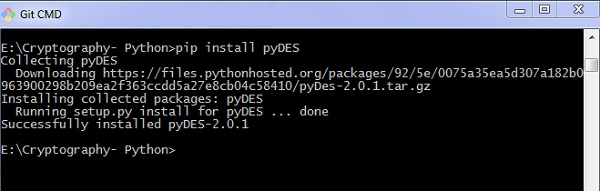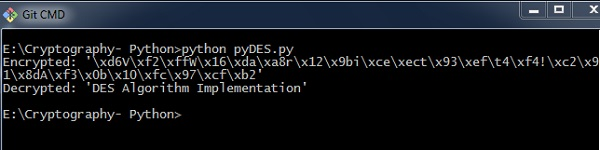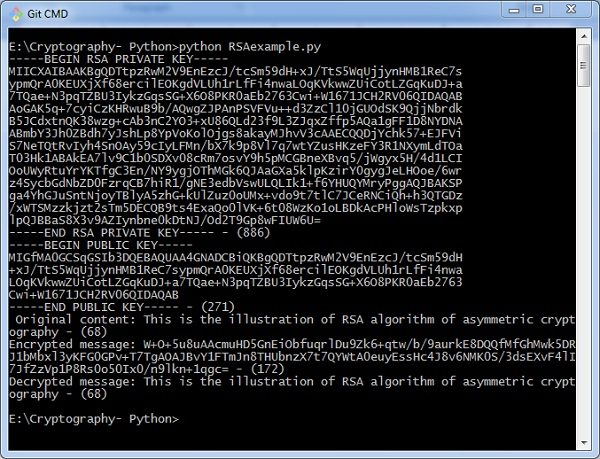# Symmetric and Asymmetric Cryptography

In this chapter, let us discuss in detail about symmetric and asymmetric cryptography.

## Symmetric Cryptography

In this type, the encryption and decryption process uses the same key. It is also called as secret key cryptography. The main features of symmetric cryptography are as follows −

• It is simpler and faster.
• The two parties exchange the key in a secure way.

### Drawback

The major drawback of symmetric cryptography is that if the key is leaked to the intruder, the message can be easily changed and this is considered as a risk factor.

## Data Encryption Standard (DES)

The most popular symmetric key algorithm is Data Encryption Standard (DES) and Python includes a package which includes the logic behind DES algorithm.

### Installation

The command for installation of DES package pyDES in Python is −

pip install pyDESSimple program implementation of DES algorithm is as follows −

import pyDes

data = "DES Algorithm Implementation"
d = k.encrypt(data)

print "Encrypted: %r" % d
print "Decrypted: %r" % k.decrypt(d)
assert k.decrypt(d) == data


It calls for the variable padmode which fetches all the packages as per DES algorithm implementation and follows encryption and decryption in a specified manner.

### Output

You can see the following output as a result of the code given above −## Asymmetric Cryptography

It is also called as public key cryptography. It works in the reverse way of symmetric cryptography. This implies that it requires two keys: one for encryption and other for decryption. The public key is used for encrypting and the private key is used for decrypting.

### Drawback

• Due to its key length, it contributes lower encryption speed.
• Key management is crucial.

The following program code in Python illustrates the working of asymmetric cryptography using RSA algorithm and its implementation −

from Crypto import Random
from Crypto.PublicKey import RSA
import base64

def generate_keys():
# key length must be a multiple of 256 and >= 1024
modulus_length = 256*4
publickey = privatekey.publickey()
return privatekey, publickey

def encrypt_message(a_message , publickey):
encrypted_msg = publickey.encrypt(a_message, 32)
encoded_encrypted_msg = base64.b64encode(encrypted_msg)
return encoded_encrypted_msg

def decrypt_message(encoded_encrypted_msg, privatekey):
decoded_encrypted_msg = base64.b64decode(encoded_encrypted_msg)
decoded_decrypted_msg = privatekey.decrypt(decoded_encrypted_msg)
return decoded_decrypted_msg

a_message = "This is the illustration of RSA algorithm of asymmetric cryptography"
privatekey , publickey = generate_keys()
encrypted_msg = encrypt_message(a_message , publickey)
decrypted_msg = decrypt_message(encrypted_msg, privatekey)

print "%s - (%d)" % (privatekey.exportKey() , len(privatekey.exportKey()))
print "%s - (%d)" % (publickey.exportKey() , len(publickey.exportKey()))
print " Original content: %s - (%d)" % (a_message, len(a_message))
print "Encrypted message: %s - (%d)" % (encrypted_msg, len(encrypted_msg))
print "Decrypted message: %s - (%d)" % (decrypted_msg, len(decrypted_msg))


### Output

You can find the following output when you execute the code given above −